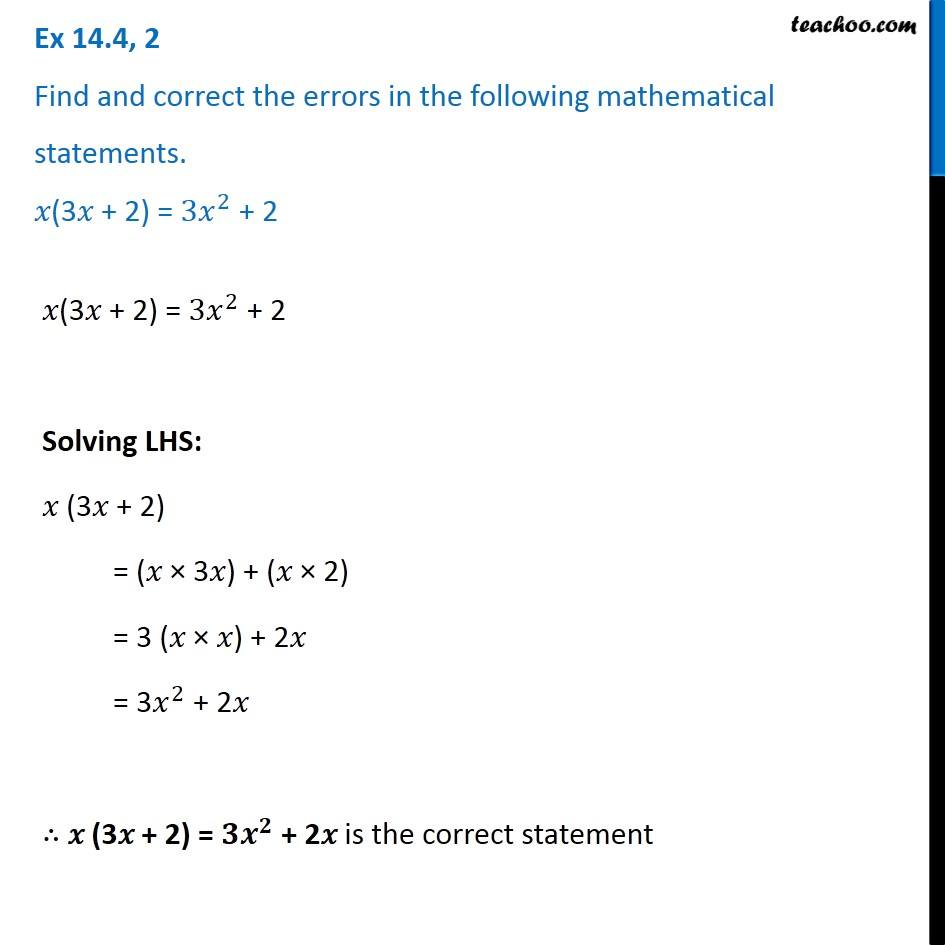1. Chapter 14 Class 8 Factorisation
2. Concept wise
3. Finding Errors

Transcript

Ex 14.4, 2 Find and correct the errors in the following mathematical statements. 𝑥(3𝑥 + 2) = 〖3𝑥〗^2 + 2 𝑥(3𝑥 + 2) = 〖3𝑥〗^2 + 2 Solving LHS: 𝑥 (3𝑥 + 2) = (𝑥 × 3𝑥) + (𝑥 × 2) = 3 (𝑥 × 𝑥) + 2𝑥 = 3𝑥^2 + 2𝑥 ∴ 𝑥 (3𝑥 + 2) = 〖𝟑𝒙〗^𝟐 + 2𝑥 is the correct statement